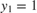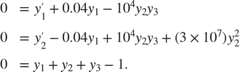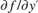# ode15i

Solve fully implicit differential equations — variable order method

## Syntax

[t,y] = ode15i(odefun,tspan,y0,yp0)
[t,y] = ode15i(odefun,tspan,y0,yp0,options)
[t,y,te,ye,ie] = ode15i(odefun,tspan,y0,yp0,options)
sol = ode15i(___)

## Description

example

[t,y] = ode15i(odefun,tspan,y0,yp0), where tspan = [t0 tf], integrates the system of differential equations $f\left(t,y,y\text{'}\right)=0$ from t0 to tf with initial conditions y0 and yp0. Each row in the solution array y corresponds to a value returned in column vector t.

example

[t,y] = ode15i(odefun,tspan,y0,yp0,options) also uses the integration settings defined by options, which is an argument created using the odeset function. For example, use the AbsTol and RelTol options to specify absolute and relative error tolerances, or the Jacobian option to provide the Jacobian matrix.
[t,y,te,ye,ie] = ode15i(odefun,tspan,y0,yp0,options) additionally finds where functions of (t,y,y'), called event functions, are zero. In the output, te is the time of the event, ye is the solution at the time of the event, and ie is the index of the triggered event.For each event function, specify whether the integration is to terminate at a zero and whether the direction of the zero crossing matters. Do this by setting the 'Events' property to a function, such as myEventFcn or @myEventFcn, and creating a corresponding function: [value,isterminal,direction] = myEventFcn(t,y,yp). For more information, see ODE Event Location.
sol = ode15i(___) returns a structure that you can use with deval to evaluate the solution at any point on the interval [t0 tf]. You can use any of the input argument combinations in previous syntaxes.

## Examples

collapse all

Calculate consistent initial conditions and solve an implicit ODE with ode15i.

Weissinger's equation is

${\mathrm{ty}}^{2}{\left({\mathit{y}}^{\prime }\right)}^{3}-{\mathit{y}}^{3}{\left({\mathit{y}}^{\prime }\right)}^{2}+\mathit{t}\left({\mathit{t}}^{2}+1\right){\mathit{y}}^{\prime }-{\mathit{t}}^{2}\mathit{y}=0$.

Since the equation is in the generic form $\mathit{f}\left(\mathit{t},\mathit{y},{\mathit{y}}^{\prime }\right)=0$, you can use the ode15i function to solve the implicit differential equation.

Code Equation

To code the equation in a form suitable for ode15i, you need to write a function with inputs for $\mathit{t}$, $\mathit{y}$, and ${\mathit{y}}^{\prime }$ that returns the residual value of the equation. The function @weissinger encodes this equation. View the function file.

type weissinger
function res = weissinger(t,y,yp) %WEISSINGER Evaluate the residual of the Weissinger implicit ODE % % See also ODE15I. % Jacek Kierzenka and Lawrence F. Shampine % Copyright 1984-2014 The MathWorks, Inc. res = t*y^2 * yp^3 - y^3 * yp^2 + t*(t^2 + 1)*yp - t^2 * y; 

Calculate Consistent Initial Conditions

The ode15i solver requires consistent initial conditions, that is, the initial conditions supplied to the solver must satisfy

$\mathit{f}\left({\mathit{t}}_{0},\mathit{y},{\mathit{y}}^{\prime }\right)=0$.

Since it is possible to supply inconsistent initial conditions, and ode15i does not check for consistency, it is recommended that you use the helper function decic to compute such conditions. decic holds some specified variables fixed and computes consistent initial values for the unfixed variables.

In this case, fix the initial value $\mathit{y}\left({\mathit{t}}_{0}\right)=\sqrt{\frac{3}{2}}$ and let decic compute a consistent initial value for the derivative ${\mathit{y}}^{\prime }\left({\mathit{t}}_{0}\right)$, starting from an initial guess of ${\mathit{y}}^{\prime }\left({\mathit{t}}_{0}\right)=0$.

t0 = 1; y0 = sqrt(3/2); yp0 = 0; [y0,yp0] = decic(@weissinger,t0,y0,1,yp0,0)
y0 = 1.2247 
yp0 = 0.8165 

Solve Equation

Use the consistent initial conditions returned by decic with ode15i to solve the ODE over the time interval $\left[1\text{\hspace{0.17em}}10\right]$.

[t,y] = ode15i(@weissinger,[1 10],y0,yp0);

Plot Results

The exact solution of this ODE is

$\mathit{y}\left(\mathit{t}\right)=\sqrt{{\mathit{t}}^{2}+\frac{1}{2}}$.

Plot the numerical solution y computed by ode15i against the analytical solution ytrue.

ytrue = sqrt(t.^2 + 0.5); plot(t,y,'*',t,ytrue,'-o') legend('ode15i', 'exact')This example reformulates a system of ODEs as a fully implicit system of differential algebraic equations (DAEs). The Robertson problem coded by hb1ode.m is a classic test problem for programs that solve stiff ODEs. The system of equations ishb1ode solves this system of ODEs to steady state with the initial conditions,, and. But the equations also satisfy a linear conservation law,In terms of the solution and initial conditions, the conservation law isThe problem can be rewritten as a system of DAEs by using the conservation law to determine the state of. This reformulates the problem as the implicit DAE systemThe function robertsidae encodes this DAE system.

function res = robertsidae(t,y,yp) res = [yp(1) + 0.04*y(1) - 1e4*y(2)*y(3); yp(2) - 0.04*y(1) + 1e4*y(2)*y(3) + 3e7*y(2)^2; y(1) + y(2) + y(3) - 1]; 

The full example code for this formulation of the Robertson problem is available in ihb1dae.m.

Set the error tolerances and the value of.

options = odeset('RelTol',1e-4,'AbsTol',[1e-6 1e-10 1e-6], ... 'Jacobian',{[],[1 0 0; 0 1 0; 0 0 0]}); 

Use decic to compute consistent initial conditions from guesses. Fix the first two components of y0 to get the same consistent initial conditions as found by ode15s in hb1dae.m, which formulates this problem as a semi-explicit DAE system.

y0 = [1; 0; 1e-3]; yp0 = [0; 0; 0]; [y0,yp0] = decic(@robertsidae,0,y0,[1 1 0],yp0,[],options); 

Solve the system of DAEs using ode15i.

tspan = [0 4*logspace(-6,6)]; [t,y] = ode15i(@robertsidae,tspan,y0,yp0,options); 

Plot the solution components. Since the second solution component is small relative to the others, multiply it by 1e4 before plotting.

y(:,2) = 1e4*y(:,2); semilogx(t,y) ylabel('1e4 * y(:,2)') title('Robertson DAE problem with a Conservation Law, solved by ODE15I')## Input Arguments

collapse all

Functions to solve, specified as a function handle that defines the functions to be integrated.

The function f = odefun(t,y,yp), for a scalar t and column vectors y and yp, must return a column vector f of data type single or double that corresponds to $f\left(t,y,y\text{'}\right)$. odefun must accept the three inputs for t, y, and yp even if one of the inputs is not used in the function.

For example, to solve $y\text{'}-y=0$, use this function.

function f = odefun(t,y,yp) f = yp - y; end

For a system of equations, the output of odefun is a vector. Each equation becomes an element in the solution vector. For example, to solve

$\begin{array}{l}y{\text{'}}_{1}-{y}_{2}=0\\ y{\text{'}}_{2}+1=0\text{\hspace{0.17em}},\end{array}$

use this function.

function dy = odefun(t,y,yp) dy = zeros(2,1); dy(1) = yp(1)-y(2); dy(2) = yp(2)+1; end

For information on how to provide additional parameters to the function odefun, see Parameterizing Functions.

Example: @myFcn

Data Types: function_handle

Interval of integration, specified as a vector. At minimum, tspan must be a two element vector [t0 tf] specifying the initial and final times. To obtain solutions at specific times between t0 and tf, use a longer vector of the form [t0,t1,t2,...,tf]. The elements in tspan must be all increasing or all decreasing.

The solver imposes the initial conditions given by y0 at the initial time tspan(1), then integrates from tspan(1) to tspan(end):

• If tspan has two elements, [t0 tf], then the solver returns the solution evaluated at each internal integration step within the interval.

• If tspan has more than two elements [t0,t1,t2,...,tf], then the solver returns the solution evaluated at the given points. However, the solver does not step precisely to each point specified in tspan. Instead, the solver uses its own internal steps to compute the solution, then evaluates the solution at the requested points in tspan. The solutions produced at the specified points are of the same order of accuracy as the solutions computed at each internal step.

Specifying several intermediate points has little effect on the efficiency of computation, but for large systems it can affect memory management.

The values of tspan are used by the solver to calculate suitable values for InitialStep and MaxStep:

• If tspan contains several intermediate points [t0,t1,t2,...,tf], then the specified points give an indication of the scale for the problem, which can affect the value of InitialStep used by the solver. Therefore, the solution obtained by the solver might be different depending on whether you specify tspan as a two-element vector or as a vector with intermediate points.

• The initial and final values in tspan are used to calculate the maximum step size MaxStep. Therefore, changing the initial or final values in tspan could lead to the solver using a different step sequence, which might change the solution.

Example: [1 10]

Example: [1 3 5 7 9 10]

Data Types: single | double

Initial conditions for y, specified as a vector. y0 must be the same length as the vector output of odefun, so that y0 contains an initial condition for each equation defined in odefun.

The initial conditions for y0 and yp0 must be consistent, meaning that $f\left({t}_{0},{y}_{0},y{\text{'}}_{0}\right)=0$. Use the decic function to compute consistent initial conditions close to guessed values.

Data Types: single | double

Initial conditions for y’, specified as a column vector. yp0 must be the same length as the vector output of odefun, so that yp0 contains an initial condition for each variable defined in odefun.

The initial conditions for y0 and yp0 must be consistent, meaning that $f\left({t}_{0},{y}_{0},y{\text{'}}_{0}\right)=0$. Use the decic function to compute consistent initial conditions close to guessed values.

Data Types: single | double

Option structure, specified as a structure array. Use the odeset function to create or modify the option structure.

See Summary of ODE Options for a list of which options are compatible with each ODE solver.

Example: options = odeset('RelTol',1e-5,'Stats','on','OutputFcn',@odeplot) specifies a relative error tolerance of 1e-5, turns on the display of solver statistics, and specifies the output function @odeplot to plot the solution as it is computed.

Data Types: struct

## Output Arguments

collapse all

Evaluation points, returned as a column vector.

• If tspan contains two elements [t0 tf], then t contains the internal evaluation points used to perform the integration.

• If tspan contains more than two elements, then t is the same as tspan.

Solutions, returned as an array. Each row in y corresponds to the solution at the value returned in the corresponding row of t.

Time of events, returned as a column vector. The event times in te correspond to the solutions returned in ye, and ie specifies which event occurred.

Solution at time of events, returned as an array. The event times in te correspond to the solutions returned in ye, and ie specifies which event occurred.

Index of triggered event function, returned as a column vector. The event times in te correspond to the solutions returned in ye, and ie specifies which event occurred.

Structure for evaluation, returned as a structure array. Use this structure with the deval function to evaluate the solution at any point in the interval [t0 tf]. The sol structure array always includes these fields:

Structure FieldDescription

sol.x

Row vector of the steps chosen by the solver.

sol.y

Solutions. Each column sol.y(:,i) contains the solution at time sol.x(i).

sol.solver

Solver name.

Additionally, if you specify the Events option of odeset and events are detected, then sol also includes these fields:

Structure FieldDescription

sol.xe

Points when events occurred. sol.xe(end) contains the exact point of a terminal event, if any.

sol.ye

Solutions that correspond to events in sol.xe.

sol.ie

Indices into the vector returned by the function specified in the Events option. The values indicate which event the solver detected.

## Tips

• Providing the Jacobian matrix to ode15i is critical for reliability and efficiency. Alternatively, if the system is large and sparse, then providing the Jacobian sparsity pattern also assists the solver. In either case, use odeset to pass in the matrices using the Jacobian or JPattern options.

## Algorithms

ode15i is a variable-step, variable-order (VSVO) solver based on the backward differentiation formulas (BDFs) of orders 1 to 5. ode15i is designed to be used with fully implicit differential equations and index-1 differential algebraic equations (DAEs). The helper function decic computes consistent initial conditions that are suitable to be used with ode15i .

 Lawrence F. Shampine, “Solving 0 = F(t, y(t), y′(t)) in MATLAB,” Journal of Numerical Mathematics, Vol.10, No.4, 2002, pp. 291-310.